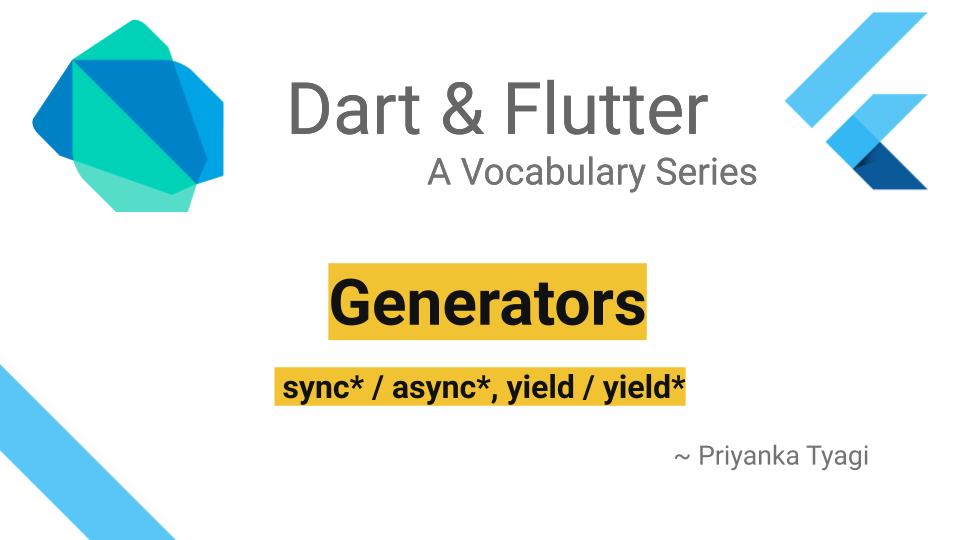# Dart Generators

Posted on June 07, 2020 in Dart# Introduction

Dart generator functions are used to generate sequence of values on-demand lazily. Such value sequence can be generated either synchronously or asynchronously. There are two types of built-in generator functions available to support both scenarios:

• Synchronous Generator: Synchronous generator function returns an Iterable object. That means first the values are generated and then returned lazily on-demand by the function.

Iterable: A collection of values, or "elements", that can be accessed sequentially.

• Asynchronous Generator: Asynchronous generator function returns a Stream object. The sequence of values is generated on demand as they become available.

Stream: A source of asynchronous data events.

Let's understand generator functions with help of an example. We'll generate numbers starting from a given number say 5 until 0, using generator functions. We'll observe both ways (asynchronous and synchronous generators) to create this number sequence.

# Using `sync*` - Synchronous Generator

The function `Iterable<int> countDownFromSync(int num) sync*` takes a number as `num`, and sends out all numbers starting from `num` until 0. The synchronous generator function is marked with `sync*`. The values are returned using `yield` keyword. The iterable `sequence` receives the number sequence and print each number using `for` loop. This number sequence actually is not generated until it has been accessed by `for` loop.

main1():

``````void main1() {
print("Getting CountDown Iterable [sync* + yield]");
Iterable<int> sequence = countDownFromSync(5);

print("Starting...");

for (int value in sequence) {
print(value);
}
print("DONE");
}

//sync*
Iterable<int> countDownFromSync(int num) sync* {
while (num > 0) {
yield num--;
}
}
``````

Output:

The `sync*` helps to generate values in a synchronous manner. Note that `Starting...` message is printed before for loop's execution. The `DONE` message is executed at last as well.

``````Getting CountDown Iterable [sync* + yield]
Starting...
5
4
3
2
1
DONE
``````

# Using `async*` - Asynchronous Generator

The function `Stream<int> countDownFromAsync(int num) async*` takes a number as `num`, and deliver number sequence starting from `num` until 0. The asynchronous generator function is marked with `async*`. The values are returned using `yield` keyword. The stream `sequence` receives the number sequence. Its values can be accessed as soon as it got started listening upon.

main2():

``````void main2() {
print("Getting CountDown Stream [async* + yield]");
Stream<int> sequence = countDownFromAsync(5);

print("Starting...");

sequence.listen((int value) {
print(value);
});
print("DONE");
}

//async*
Stream<int> countDownFromAsync(int num) async* {
while (num > 0) {
yield num--;
}
}
``````

Output:

The `async*` helps to generate values in an asynchronous manner. Note that `Starting...` and `DONE` messages/setup are printed before actual stream's values are printed. The values are printed as they become available after the setup code.

``````Getting CountDown Stream [async* + yield]
Starting...
DONE
5
4
3
2
1
``````

# Using `sync* + yield*` - Recursive Synchronous Generator

When generator functions are used recursively, `yield*` is used to mark such recursive function calls. This example shows how to use generator functions recursively. You'll notice the same output as for the non-recursive implementation. The keyword `yield*` is used for the function that's called recursively.

``````void main3() {
print("Getting CountDown Iterable [sync* + yield*]");
Iterable<int> sequence = countDownFromSyncRecursive(5);

print("Starting...");

for (int value in sequence) {
print(value);
}
print("DONE");
}

//sync* + yield* for recursive functions
Iterable<int> countDownFromSyncRecursive(int num) sync* {
if (num > 0) {
yield num;

yield* countDownFromSyncRecursive(num - 1);
}
}
``````

Output:

``````Getting CountDown Iterable [sync* + yield*]
Starting...
5
4
3
2
1
DONE
``````

# Using `async* + yield*` - Recursive Asynchronous Generator

This is an example of using an asynchronous generator function recursively. It also has the same output as its non-recursive counter-part.

``````void main4() {
print("Getting CountDown Stream [async* + yield*]");
Stream<int> sequence = countDownFromAsyncRecursive(5);

print("Starting...");

sequence.listen((int value) {
print(value);
});
print("DONE");
}

//async* + yield* for recursive functions
Stream<int> countDownFromAsyncRecursive(int num) async* {
if (num > 0) {
yield num;

yield* countDownFromAsyncRecursive(num - 1);
}
}
``````

Output:

``````Getting CountDown Stream [async* + yield*]
Starting...
DONE
5
4
3
2
1
``````

# Summary

In this article, we saw how to use Dart's generator function to produce an on-demand sequence of values synchronously and asynchronously in an iterative and recursive manner.

That's it for this article. Check out the Dart Vocabulary Series for other Dart stuff.

# Source Code

Please checkout the source code at Github here

Happy Darting :)